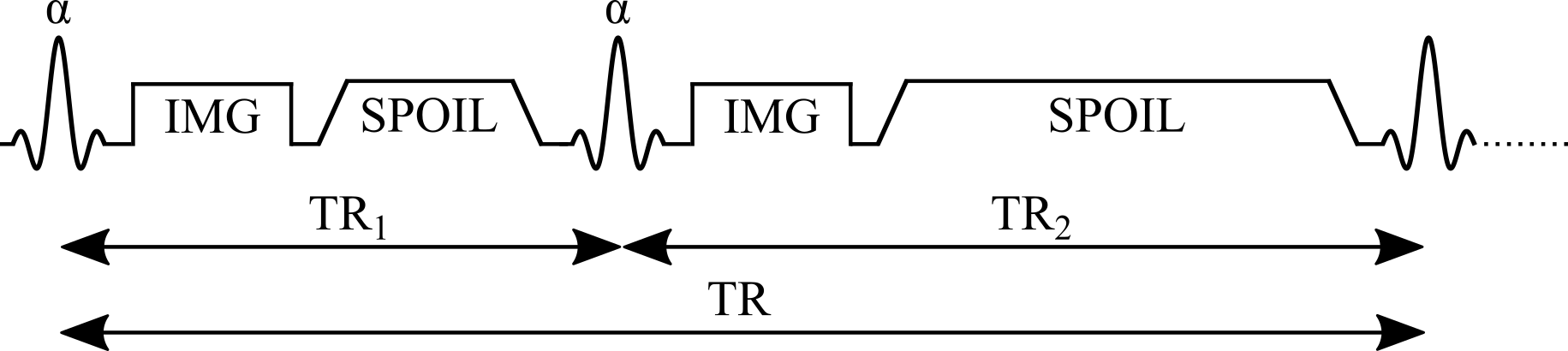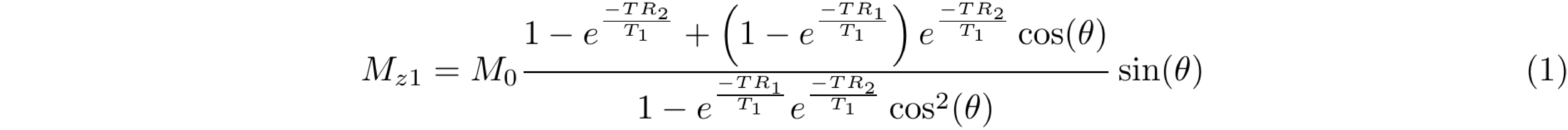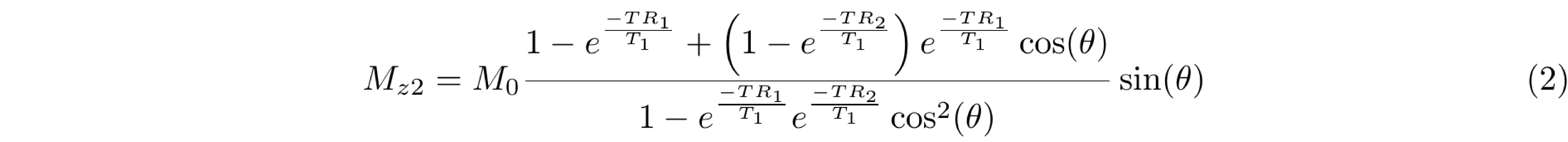Display content:

In :
% **Blog post code introduction**
%
% Congrats on activating the "All cells" option in this interactive blog post =D
%
% Below, several new HTML blocks have appears prior to the figures, displaying the Octave/MATLAB code that was used to generate the figures in this blog post.
%
% If you want to reproduce the data on your own local computer, you simply need to have qMRLab installed in your Octave/MATLAB path and run the "startup.m" file, as is shown below.
%
% If you want to get under the hood and modify the code right now, you can do so in the Jupyter Notebook of this blog post hosted on MyBinder. The link to it is in the introduction above.

In :

# Actual flip-angle imaging B1 mapping

Transmit radiofrequency field maps (B1+, or B1 for short) are used in diverse applications in MRI including: the study of electrical properties in tissues in vivo (Sled & Pike 1998; Katscher et al. 2009), specific absorption rate (SAR) calculations (Ibrahim et al. 2001), the calibration of quantitative T1 (Deoni 2007; Boudreau et al. 2017) and T2 (Sled and Pike 2000) maps, better parameter estimation from magnetization transfer measurements (Ropele et al. 2005; Boudreau et al. 2018), B1 shimming to improve image quality at whole-body ultra high fields (van den Bergen et al. 2007), or quality control of RF coils (Yarnykh 2007). Several B1 mapping techniques have been developed, and they can be broadly divided as magnitude-based and phase-based methods. The double angle method (DAM) is a saturation-recovery magnitude-based method that takes the ratio of the signal intensity of two magnitude images measured with different excitation flip angles (Insko & Bolinger 1993; Stollberger and Wach 1996). The Bloch-Siegert shift technique is a rapid phase-based method that encodes the B1 information into phase signal (Sacolick et al. 2010). The actual flip-angle imaging (AFI) is a magnitude-based B1 mapping method that consists of a 3D acquisition that benefits from good anatomical coverage. In addition, this technique allows the acquisitions of whole-body (~7 min) and brain (~3 min) B1 maps leading to a feasible implementation in clinics (Yarnykh 2004; Yarnykh 2007). On the other hand, the AFI pulse sequence has certain constraints that need to be considered for this B1 mapping method to be widely deployed. Some of the limitations include the use of spoiler gradients that can give rise to prohibitive SAR values (Sacolick et al. 2010), and the pulse sequence modifications on the MRI machine to implement the AFI method.

In this blog post, we will focus on presenting details about the AFI B1 mapping method. We will cover signal modeling, data fitting, the benefits and the pitfalls of the technique. The figures are generated using the qMRLab module for this method.

## Signal Modelling

The pulse sequence of the AFI method (Figure 1) is composed of two identical RF pulses and two different delays (TR1 < TR2). After each RF pulse, the signal intensity is acquired followed by a spoiler to destroy the residual transverse magnetization next to the following RF pulse. This method implements a pulsed steady-state signal with a gradient-echo acquisition, thus preventing the use of long repetition times (Yarnykh 2007). It has been demonstrated that if the delays TR1 and TR2 are sufficiently short (e.g. TR1/TR2 = 20 ms/100 ms), and the transverse magnetization is completely spoiled, the ratio of signal intensities (r = S2/S1) depends on the flip angle of applied pulses and is highly insensitive to T1 (Yarnykh 2007).

Figure 1. Simplified pulse sequence diagram of an actual flip-angle imaging (AFI) pulse sequence with a gradient echo readout. TR1: repetition time 1, TR2: repetition time 2, θ: excitation flip angle for the measurement, IMG: image acquisition (k-space readout), SPOIL: spoiler gradient.The magnetization of an AFI experiment can be modeled under steady-state conditions by the implementation of a fast repetition of the sequence (TR1 < TR2 < T1). The solution of the Bloch equations for the AFI method is given by Equations 1 and 2 that represent the longitudinal magnetization before the application of the RF pulses:Mz1,2 is the longitudinal magnetization of both pulses, M0 is the magnetization at thermal equilibrium, TR1 is the delay time after the first pulse, TR2 is the delay time after the second identical pulse (Figure 1), and θ is the excitation flip angle. The steady-state longitudinal magnetization Mz curves for different T1 values for a range of θn and TR values are shown in Figure 2.

Figure 2. Longitudinal magnetization before the first radiofrequency pulse (Equation 1, solid lines) and before the second identical pulse (Equation 2, dashed lines) for three different T1 values.

In :
%% MATLAB/OCTAVE CODE
% Adds qMRLab to the path of the environment

cd ../qMRLab
startup

warning: function /home/jovyan/work/t1_notebooks/qMRLab/External/NODDI_toolbox_v1.0/ParforProgMonv2/example.m shadows a core library function
warning: called from
startup at line 2 column 1
-----------------------------------------------
Release v2.4.2
Hawdy developer!  The version specified in version.txt is ahead of the latest published release v2.4.1.
Please do not forget pushing a new commit tag upon merge or publish the new release.
-----------------------------------------------

In :
%% MATLAB/OCTAVE CODE
% Code used to generate the data required for Figure 2 of the blog post

clear all

%% Setup parameters
% All times are in milliseconds
% All flip angles are in degrees

TR1_range = 5:5:50;
n = 5;
TR2_range = n*TR1_range;

params.EXC_FA = 1:90;

%% Calculate signals
%
% To see all the options available, run help b1_afi.analytical_solution

for ii = 1:length(TR1_range)
params.TR1 = TR1_range(ii);
params.TR2 = TR2_range(ii);

% White matter
params.T1 = 900; % in milliseconds
[Mz1, Mz2] = b1_afi.analytical_solution(params);
signal1_WM(ii,:) = Mz1;
signal2_WM(ii,:) = Mz2;

% Grey matter
params.T1 = 1500;  % in milliseconds
[Mz1, Mz2] = b1_afi.analytical_solution(params);
signal1_GM(ii,:) = Mz1;
signal2_GM(ii,:) = Mz2;

% CSF
params.T1 = 4000;  % in milliseconds
[Mz1, Mz2] = b1_afi.analytical_solution(params);
signal1_CSF(ii,:) = Mz1;
signal2_CSF(ii,:) = Mz2;
end

In :
In :
# PYTHON CODE

init_notebook_mode(connected=True)

data1_1 = [dict(
visible = False,
line=dict(color='royalblue'),
x = params["EXC_FA"],
y = abs(np.squeeze(np.asarray(signal1_WM[ii]))),
name = 'S<sub>1</sub>: T<sub>1</sub> = 0.9 s (White Matter)',
text = 'S<sub>1</sub>: T<sub>1</sub> = 0.9 s (White Matter)',
hoverinfo = 'x+y+text') for ii in range(len(TR1_range))]

data1_1['visible'] = True

data1_2 = [dict(
visible = False,
line=dict(color='royalblue', dash='dash'),
x = params["EXC_FA"],
y = abs(np.squeeze(np.asarray(signal2_WM[ii]))),
name = 'S<sub>2</sub>: T<sub>1</sub> = 0.9 s (White Matter)',
text = 'S<sub>2</sub>: T<sub>1</sub> = 0.9 s (White Matter)',
hoverinfo = 'x+y+text') for ii in range(len(TR1_range))]

data1_2['visible'] = True

data2_1 = [dict(
visible = False,
line=dict(color='firebrick'),
x = params["EXC_FA"],
y = abs(np.squeeze(np.asarray(signal1_GM[ii]))),
name = 'S<sub>1</sub>: T<sub>1</sub> = 1.5 s (Grey Matter)',
text = 'S<sub>1</sub>: T<sub>1</sub> = 1.5 s (Grey Matter)',
hoverinfo = 'x+y+text') for ii in range(len(TR1_range))]

data2_1['visible'] = True

data2_2 = [dict(
visible = False,
line=dict(color='firebrick', dash='dash'),
x = params["EXC_FA"],
y = abs(np.squeeze(np.asarray(signal2_GM[ii]))),
name = 'S<sub>2</sub>: T<sub>1</sub> = 1.5 s (Grey Matter)',
text = 'S<sub>2</sub>: T<sub>1</sub> = 1.5 s (Grey Matter)',
hoverinfo = 'x+y+text') for ii in range(len(TR1_range))]

data2_2['visible'] = True

data3_1 = [dict(
visible = False,
line=dict(color='orange'),
x = params["EXC_FA"],
y = abs(np.squeeze(np.asarray(signal1_CSF[ii]))),
name = 'S<sub>1</sub>: T<sub>1</sub> = 4.0 s (Cerebrospinal Fluid)',
text = 'S<sub>1</sub>: T<sub>1</sub> = 4.0 s (Cerebrospinal Fluid)',
hoverinfo = 'x+y+text') for ii in range(len(TR1_range))]

data3_1['visible'] = True

data3_2 = [dict(
visible = False,
line=dict(color='orange', dash='dash'),
x = params["EXC_FA"],
y = abs(np.squeeze(np.asarray(signal2_CSF[ii]))),
name = 'S<sub>2</sub>: T<sub>1</sub> = 4.0 s (Cerebrospinal Fluid)',
text = 'S<sub>2</sub>: T<sub>1</sub> = 4.0 s (Cerebrospinal Fluid)',
hoverinfo = 'x+y+text') for ii in range(len(TR1_range))]

data3_2['visible'] = True

data = data1_1 + data1_2 + data2_1 +data2_2 + data3_1 + data3_2

steps = []
for i in range(len(TR1_range)):
step = dict(
method = 'restyle',
args = ['visible', [False] * len(data1_1)],
label = str(TR1_range[i])
)
step['args'][i] = True # Toggle i'th trace to "visible"
steps.append(step)

sliders=[
dict(
x = 0,
y = -0.09,
active = 3,
currentvalue = {"prefix": "TR1 value (ms): <b>"},
pad = {"t": 50, "b": 10},
steps = steps)]

layout = go.Layout(
width=650,
height=520,
margin=go.layout.Margin(
l=80,
r=40,
b=60,
t=10,
),
annotations=[
dict(
x=0.5004254919715793,
y=-0.18,
showarrow=False,
text='Excitation Flip Angle (°)',
font=dict(
family='Times New Roman',
size=22
),
xref='paper',
yref='paper'
),
dict(
x=-0.15,
y=0.5,
showarrow=False,
text='Long. Magnetization (M<sub>z</sub>)',
font=dict(
family='Times New Roman',
size=22
),
textangle=-90,
xref='paper',
yref='paper'
),
dict(
x=-0.007,
y=-0.25,
showarrow=False,
text='TR<sub>2</sub> = 5TR<sub>1</sub>',
font=dict(
family='Times New Roman',
size=12
),
xref='paper',
yref='paper'
),
],
xaxis=dict(
autorange=False,
range=[0, params['EXC_FA'][-1]],
showgrid=False,
linecolor='black',
linewidth=2
),
yaxis=dict(
autorange=True,
showgrid=False,
linecolor='black',
linewidth=2
),
legend=dict(
x=0.5,
y=0.9,
traceorder='normal',
font=dict(
family='Times New Roman',
size=12,
color='#000'
),
bordercolor='#000000',
borderwidth=2
),
sliders=sliders
)

fig = dict(data=data, layout=layout)

iplot(fig, filename = 'basic-line', config = config)


The analytical solution of the Bloch equations in a steady-state experiment (Equation 1 and Equation 2) makes several assumptions leading to practical challenges. First, it is assumed that the longitudinal magnetization has reached a steady state after a sufficiently large number of repetition times (TR), and that the transverse magnetization is perfectly spoiled prior to each pulse. To explore these properties, a numerical approach known as Bloch simulations is used to estimate the signal from an MRI experiment given a set of sequence parameters. Here, the Bloch simulations allow us to estimate the magnetization using a different number of sequence repetitions, and look at a special case when the steady-state is not achieved (due to a small number of sequence repetitions). As can be seen in Figure 3, the number of repetitions required to reach a steady-state depends on T1 and the flip angle.

Figure 3. Signal 1 (blue) and Signal 2 (red) curves simulated using Bloch simulations (solid lines) for a number of repetitions ranging from 1 to 150, plotted against the ideal case (Equations 1 and 2 – dashed lines). Simulation details: TR1 = 20 ms, TR2 = 100 ms, T1 = 900 ms, 100 spins. Ideal spoiling was used for this set of Bloch simulations (transverse magnetization was set to 0 at the end of each TR1,2).

In :
%% MATLAB/OCTAVE CODE
% Code used to generate the data required for Figure 3 of the blog post

clear all

%% Setup parameters
% All times are in milliseconds
% All flip angles are in degrees

% White matter
params.T1 = 900; % in milliseconds
params.T2 = 10000;
params.TR1 = 20;
params.TR2 = 100;
params.TE = 5;
params.EXC_FA = 1:90;
Nex_range = 1:1:150;

%% Calculate signals
% This results were precomputed. Uncomment the lines below to run the simulation.
signal1_blochsim = signal1_bloch.signal1_blochsim;
signal2_blochsim = signal2_bloch.signal2_blochsim;
signal1_analytical = signal1_analytical.signal1_analytical;
signal2_analytical = signal2_analytical.signal2_analytical;

%{
% Bloch simulations
% To see all the options available, run help b1_afi.analytical_solution

for ii = 1:length(Nex_range)
params.Nex = Nex_range(ii);

[Mz1, Mz2] = b1_afi.analytical_solution(params);
signal1_analytical(ii,:) = Mz1;
signal2_analytical(ii,:) = Mz2;

[~, Msig1, Msig2] = b1_afi.bloch_sim(params);
signal1_blochsim(ii,:) = abs(complex(Msig1));
signal2_blochsim(ii,:) = abs(complex(Msig2));
end
%}

In :
In :
# PYTHON CODE

init_notebook_mode(connected=True)

data1_1 = [dict(
visible = False,
line=dict(color='royalblue', dash='dash'),
x = params["EXC_FA"],
y = abs(np.squeeze(np.asarray(signal1_analytical[ii]))),
name = 'S<sub>1</sub>: Analytical Solution',
text = 'S<sub>1</sub>: Analytical Solution',
hoverinfo = 'x+y+text') for ii in range(len(Nex_range))]

data1_1['visible'] = True

data1_2 = [dict(
visible = False,
line=dict(color='firebrick', dash='dash'),
x = params["EXC_FA"],
y = abs(np.squeeze(np.asarray(signal2_analytical[ii]))),
name = 'S<sub>2</sub>: Analytical Solution',
text = 'S<sub>2</sub>: Analytical Solution',
hoverinfo = 'x+y+text') for ii in range(len(Nex_range))]

data1_2['visible'] = True

data2_1 = [dict(
visible = False,
line=dict(color='royalblue'),
x = params["EXC_FA"],
y = abs(np.squeeze(np.asarray(signal1_blochsim[ii]))),
name = 'S<sub>1</sub>: Bloch Simulation',
text = 'S<sub>1</sub>: Bloch Simulation',
hoverinfo = 'x+y+text') for ii in range(len(Nex_range))]

data2_1['visible'] = True

data2_2 = [dict(
visible = False,
line=dict(color='firebrick'),
x = params["EXC_FA"],
y = abs(np.squeeze(np.asarray(signal2_blochsim[ii]))),
name = 'S<sub>2</sub>: Bloch Simulation',
text = 'S<sub>2</sub>: Bloch Simulation',
hoverinfo = 'x+y+text') for ii in range(len(Nex_range))]

data2_2['visible'] = True

data = data1_1 + data2_1 + data1_2 + data2_2

steps = []
for i in range(len(Nex_range)):
step = dict(
method = 'restyle',
args = ['visible', [False] * len(data1_1)],
label = str(Nex_range[i])
)
step['args'][i] = True # Toggle i'th trace to "visible"
steps.append(step)

sliders = [dict(
x = 0,
y = -0.02,
active = 5,
currentvalue = {"prefix": "n<sup>th</sup> TR1-TR2: <b>"},
pad = {"t": 50, "b": 10},
steps = steps)]

layout = go.Layout(
width=580,
height=450,
margin=go.layout.Margin(
l=80,
r=40,
b=60,
t=10,
),
annotations=[
dict(
x=0.5004254919715793,
y=-0.18,
showarrow=False,
text='Excitation Flip Angle (°)',
font=dict(
family='Times New Roman',
size=22
),
xref='paper',
yref='paper'
),
dict(
x=-0.15,
y=0.5,
showarrow=False,
text='Signal',
font=dict(
family='Times New Roman',
size=22
),
textangle=-90,
xref='paper',
yref='paper'
),
],
xaxis=dict(
autorange=False,
range=[0, params['EXC_FA'][-1]],
showgrid=False,
linecolor='black',
linewidth=2
),
yaxis=dict(
autorange=True,
showgrid=False,
linecolor='black',
linewidth=2
),
legend=dict(
x=0.5,
y=0.9,
traceorder='normal',
font=dict(
family='Times New Roman',
size=12,
color='#000'
),
bordercolor='#000000',
borderwidth=2
),
sliders=sliders
)

fig = dict(data=data, layout=layout)

iplot(fig, filename = 'basic-line', config = config)